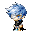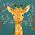## Rachille's Proportion Problems

http://en.wikipedia.org/wiki/Lemon

1)To make lemonade, you need 6 lemons and 8 cups of water. How many lemons would you need if you have 24 cups of water?

Let x be the amount of lemons for 24 cups of water.
Word ratio, ratio table, find x (cross product)
You would need 18 lemons to make lemonade with 24 cups of water.

http://commons.wikimedia.org/wiki/File:Feet_on_scale.jpg

2) The ratio of an objects weight on Earth to its weight on the moon is 6:1. the first person to walk on the moon was Neil Armstrong. He weighed 165 lb on Earth. How much did he weigh on the moon?

Let x be how much Neil weighed on the moon.
Word ratio, ratio table, find x (cross product)

Neil Armstrong weighed 27.5 lb on the moon.

A Word Problem for You
*At the Copy Shoppe, 18 copies cost \$1.08. At that rate, how much will 40 copies cost?
12:51 PM

#### 3 comments :

1.This comment has been removed by the author.

2.For the word problem given to us, I got an answer of \$2.40 because:
Let x be the \$ for 40 copies
Steps: Word Ratio, Ratio Table, Find x
Model:

Copies l \$
18 \$1.08
40 x

Work:

1.08 x 40 = 18(x)
43.2 = 18x
43.2/18 = 18x/18
2.40 = x

So it cost \$2.40 for 40 copies.

Also, very neat pictures as well as the Model and Work

3.